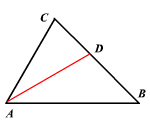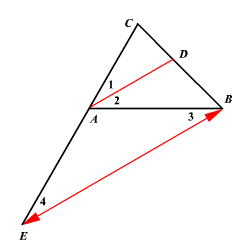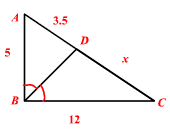Triangle Angle Bisector Theorem

An angle bisector of an angle of a triangle divides the opposite side in two segments that are proportional to the other two sides of the triangle.By the Angle Bisector Theorem,

$\frac{BD}{DC}=\frac{AB}{AC}$

Proof:

Draw $\stackrel{↔}{BE}\parallel \stackrel{↔}{AD}$ .

Extend $\stackrel{¯}{CA}$ to meet $\stackrel{↔}{BE}$ at point $E$ .By the Side-Splitter Theorem,

$\frac{CD}{DB}=\frac{CA}{AE}$ ---------( $1$ )

The angles $\angle 4\text{\hspace{0.17em}}\text{\hspace{0.17em}}\text{and}\text{\hspace{0.17em}}\text{\hspace{0.17em}}\angle 1$ are corresponding angles.

So, $\angle 4\cong \angle 1$ .

Since $\stackrel{¯}{AD}$ is a angle bisector of the angle $\angle CAB,\text{\hspace{0.17em}}\text{\hspace{0.17em}}\angle 1\cong \angle 2$ .

By the Alternate Interior Angle Theorem , $\angle 2\cong \angle 3$ .

Therefore, by transitive property, $\angle 4\cong \angle 3$ .

Since the angles $\angle 3\text{\hspace{0.17em}}\text{\hspace{0.17em}}\text{and}\text{\hspace{0.17em}}\text{\hspace{0.17em}}\angle 4$ are congruent , the triangle $\Delta ABE$ is an isosceles triangle with $AE=AB$ .

Replacing $\mathrm{AE}$ by $\mathrm{AB}$ in equation ( $1$ ),

$\frac{CD}{DB}=\frac{CA}{AB}$

Example:Find the value of $x$ .

By Triangle-Angle-Bisector Theorem,

$\frac{AB}{BC}=\frac{AD}{DC}$ .

Substitute.

$\frac{5}{12}=\frac{3.5}{x}$

Cross multiply.

$5x=42$

Divide both sides by $5$ .

$\begin{array}{l}\frac{5x}{5}=\frac{42}{5}\\ x=8.4\end{array}$

The value of $x$ is $8.4$ .